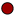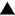Geometry section of MathBitsNotebook.comSegments, Angles and TrianglesNOTE: The re-posting of materials (in part or whole) from this site to the Internet is copyright violation and is not considered "fair use" for educators. Please read the "Terms of Use".
Topic Standards:NGMSCCSS

 LESSONS: * calculator link
Segments in Triangles (medians, altitudes, angle bisectors, perpendicular bisectors)Mid-Segments in Triangles (definition, examples, proofs of theorem)Vertical Angles (definition, examples, proofs of theorem)Sum of Interior Angles in a Triangle (examples, cut-up, various methods of proof)Exterior Angles in a Triangle (definition, examples, proof of theorem)Isosceles Triangles (definition, theorems, proofs of theorem, examples)Triangle Inequality ("in one triangle", theorems, examples)Hinge Theorem ("triangle inequaltiy in two triangles", theorems, examples)

 PRACTICE: • Practice with Segments• Practice with Segments in Triangles• Practice with MidSegments• Practice with Angles• Practice with Angles in Triangles• Practice with Isosceles Triangles• Practice with Triangle Inequality• Practice with Hinge Theorem • Pre-Proof Reasoning• Practice with Proofs (using concepts from this unit)REFRESHERS: (while these topics are mostly "review", you may also find new information here) • Segments (definition, Ruler Postulate, Segment Addition Postulate, midpoint, bisector) • All About Angles (definition, angle names, types of angles) • Pairs of Angles (adjacent, linear pair, vertical, complementary, supplementary, theorems) • Working with Angles (Angle Addition Postulate, bisect angle, angles about a pt, angles on a line) • Types of Triangles (classified by angles, classified by sides)